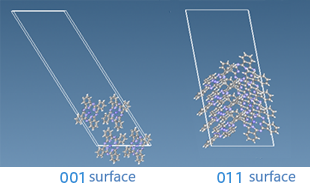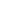Quantum Chemistry / DFT
Interface / Phase Separation / Particle Dispersion
Materials Science

# Surface Energy of Pigments

## Objectives and methods

The surface energies of the 001 and 011 planes of copper phthalocyanine (CuPc), one of the cyanide organic pigments, were calculated by SIESTA using the density functional theory and compared.

It is believed that the interfacial tension between the particles and the solvent affects the dispersibility of pigment particles in the solvent. This calculation is a basic study for this purpose.

The surface energy $$\gamma$$  was calculated from the energy difference between the bulk and surface models of the pigment model.

$$\Delta$$ = $$E_{\rm surface} - E_{\rm bulk}$$

$$\gamma$$ = $$\Delta$$ /$$2A$$

($$A$$ = surface area)

Since it is a molecular crystal, the VDW functional was used in the density functional theory calculation using SIESTA. In the $$E_{\rm surface}$$ calculation, the structural relaxation of the surface is not taken into account.

## ResultsFig. Surface structure of copper phthalocyanine

The surface energies of the 001 and 011 surfaces are 0.12 [J/m2] and 0.21 [J/m2], respectively.
It is expected that the effect of intermolecular interactions on the surface energy is stronger for the 011 surface, and the results are consistent with this expectation.

By using SIESTA, we were able to evaluate the surface energy of pigments.This website uses cookies to improve functionality and performance. If you continue browsing the site, you are giving implied consent to the use of cookies on this website. If you want to know more or refuse consent, read our Cookie Policy.

Accept